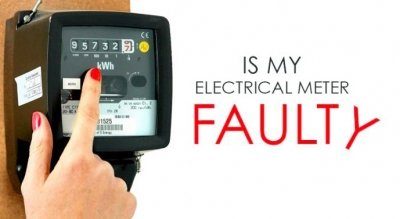## Electrical Consumption Meters – Are they accurate? How can we check?Electrical Consumption Meters – Are they accurate? How can we check?

This short series of ‘blogs’ will attempt to enlighten on the subject of electrical energy, what it is, how its measured, how much (quantum) do we use, say when boiling a mug of water in a standard kettle, what is the average electrical energy consumption per household?

The aim of the series of blog’s, is to discuss, understand and be aware of electrical consumption meters, should we trust the measurement of electrical energy our consumption meter conveys to us, and how can we check the accuracy of our electrical energy consumption meter.

Finally we shall briefly discuss the introduction to everyday ‘life’, of electrical ‘smart meters’, paying particular attention to the accuracy and security of data collated and communicated by a smart meter.

So we will begin by introducing the rudiments of electrical energy.

What is a kilo watt hour??

The kilowatt-hour (kWh, also kWHr) is a unit of energy equal to 3600 kilojoules (3.6 megajoules, a joule is also a unit of energy). The kilowatt-hour is commonly used as a billing unit for electrical energy delivered to consumers by electric utilities.

The keyword in the latter is ‘energy’, and energy is the quantitative property that must be transferred to an object, an entity in order to perform work on, or to heat.

From here on we shall discuss electrical energy yet please be aware that there are many forms of energy, i.e. mechanical, magnetic, gravitational, chemical, ionization, nuclear, chromodynamic, elastic, mechanical wave, sound wave, radiant, rest, and thermal.

Mathematically, energy (E) is expressed as power (p) (utilised, expended) over a period of time (t), i.e.

Energy (E)=power (p)× time (t) Equation 1.

So for example, if an electrical appliance utilises one (1) kilowatt of electrical power for one (1) hour then the appliance will consume one (1) kilowatt-hour (kWh, also kWHr) of electrical energy.

Further,…
If an electrical appliance utilises one (1) kilowatt of electrical power for one half (0.5) hour then the appliance will consume a half kilowatt-hour of electrical energy.

Or moreover,…
If an electrical appliance utilises one (1) kilowatt of electrical power for ten (10) minutes of one (1) hour then the appliance will consume on tenth of a kilowatt-hour of electrical energy.

In simple terms electrical power (p) is derived from the product of electrical voltage (v) and electrical current (i), which mathematically is expressed as,..

Electrical Power (p)=electrical volatge (v)× electrical current (i) Equation 2.

So for example, if a standard electrical kettle is ‘plugged’ into a standard UK domestic electrical supply, i.e. 230 volts ac (alternating current supply) and when switched on the kettle draws, say eight (8) amperes of electrical current then the power drawn is,…

230 (electrical voltage (v))× 8 (electrical current (i))= 1840 Watts=1.84 kW Equation 3.

It is important to note that in equation 3, power (p) is initially derived in ‘Watts’, which is the standard unit (SI Système international (d’unités)) of power (p), however we refer to one thousand (1000) Watts as one (1) kilo-Watt

The latter is all well and good and quite fundamental. The examples are presented assuming ‘steady state’, values, i.e. that power ,voltage, and current remain constant over time. However, in real life steady state circumstances are not the norm and hence we have to consider dynamic values, i.e. values that change with respect to time (t), i.e.
Equation 1 becomes,..

Energy (E)(t)=∫power (p) dt Equation 4.

In other words Equation 4 expresses that ‘dynamic’ electrical Energy is the cumulation of the time varying quantity of electrical power over the time period, T1 to T2.

In the next blog of the series we shall continue to review examples of the electrical power attributes of standard domestic appliances and how power varies ‘dynamically’ over time when the appliance is in use. Given understanding and appreciation of these circumstance we can then continue to discuss the energy attributes of a collection of appliances, i.e. those expected within a standard dwelling for a standard family. In short our intent is to be able to derive a ‘good’ approximation for a figure of kilowatt hours (kWHr) consumed in electrical energy by a standard dwelling which houses a standard family

Thank you Robert 🙂

Eur Ing Dr Robert Brown (Robert) is a Consultant Engineer in the fields Electrical Electronic and Control Engineering. Robert is also an accomplished professional Expert Witness having prepared and presented many court compliant reports and presented oral evidence within the High Court, Crown Courts and County Courts.